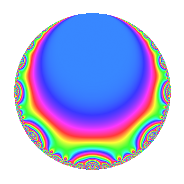Properties

 Label 8036.2.a.gLevel 8036 Weight 2 Character orbit 8036.a Self dual Yes Analytic conductor 64.168 Analytic rank 0 Dimension 2 CM No Inner twists 1

Related objects

Newspace parameters

 Level: $$N$$ = $$8036 = 2^{2} \cdot 7^{2} \cdot 41$$ Weight: $$k$$ = $$2$$ Character orbit: $$[\chi]$$ = 8036.a (trivial)

Newform invariants

 Self dual: Yes Analytic conductor: $$64.1677830643$$ Analytic rank: $$0$$ Dimension: $$2$$ Coefficient field: $$\Q(\sqrt{13})$$ Coefficient ring: $$\Z[a_1, a_2, a_3]$$ Coefficient ring index: $$1$$ Fricke sign: $$-1$$ Sato-Tate group: $\mathrm{SU}(2)$

$q$-expansion

Coefficients of the $$q$$-expansion are expressed in terms of $$\beta = \frac{1}{2}(1 + \sqrt{13})$$. We also show the integral $$q$$-expansion of the trace form.

 $$f(q)$$ $$=$$ $$q$$ $$+ ( 1 + \beta ) q^{3}$$ $$+ ( 1 + \beta ) q^{5}$$ $$+ ( 1 + 3 \beta ) q^{9}$$ $$+O(q^{10})$$ $$q$$ $$+ ( 1 + \beta ) q^{3}$$ $$+ ( 1 + \beta ) q^{5}$$ $$+ ( 1 + 3 \beta ) q^{9}$$ $$+ q^{11}$$ $$+ 5 q^{13}$$ $$+ ( 4 + 3 \beta ) q^{15}$$ $$+ ( 3 - 4 \beta ) q^{17}$$ $$+ ( 3 - 3 \beta ) q^{19}$$ $$+ ( -1 - \beta ) q^{23}$$ $$+ ( -1 + 3 \beta ) q^{25}$$ $$+ ( 7 + 4 \beta ) q^{27}$$ $$+ ( 8 + \beta ) q^{29}$$ $$+ ( -7 + 3 \beta ) q^{31}$$ $$+ ( 1 + \beta ) q^{33}$$ $$+ ( 4 - 2 \beta ) q^{37}$$ $$+ ( 5 + 5 \beta ) q^{39}$$ $$+ q^{41}$$ $$+ ( -7 - 2 \beta ) q^{43}$$ $$+ ( 10 + 7 \beta ) q^{45}$$ $$+ ( 10 - 2 \beta ) q^{47}$$ $$+ ( -9 - 5 \beta ) q^{51}$$ $$+ ( -5 + \beta ) q^{53}$$ $$+ ( 1 + \beta ) q^{55}$$ $$+ ( -6 - 3 \beta ) q^{57}$$ $$+ ( 1 - 3 \beta ) q^{59}$$ $$+ ( 1 + 4 \beta ) q^{61}$$ $$+ ( 5 + 5 \beta ) q^{65}$$ $$+ ( -5 + 3 \beta ) q^{67}$$ $$+ ( -4 - 3 \beta ) q^{69}$$ $$+ ( -3 - 4 \beta ) q^{71}$$ $$-7 q^{73}$$ $$+ ( 8 + 5 \beta ) q^{75}$$ $$+ ( -12 - 2 \beta ) q^{79}$$ $$+ ( 16 + 6 \beta ) q^{81}$$ $$+ ( -3 + 6 \beta ) q^{83}$$ $$+ ( -9 - 5 \beta ) q^{85}$$ $$+ ( 11 + 10 \beta ) q^{87}$$ $$+ ( -3 + 5 \beta ) q^{89}$$ $$+ ( 2 - \beta ) q^{93}$$ $$+ ( -6 - 3 \beta ) q^{95}$$ $$+ ( 7 - 7 \beta ) q^{97}$$ $$+ ( 1 + 3 \beta ) q^{99}$$ $$+O(q^{100})$$ $$\operatorname{Tr}(f)(q)$$ $$=$$ $$2q$$ $$\mathstrut +\mathstrut 3q^{3}$$ $$\mathstrut +\mathstrut 3q^{5}$$ $$\mathstrut +\mathstrut 5q^{9}$$ $$\mathstrut +\mathstrut O(q^{10})$$ $$2q$$ $$\mathstrut +\mathstrut 3q^{3}$$ $$\mathstrut +\mathstrut 3q^{5}$$ $$\mathstrut +\mathstrut 5q^{9}$$ $$\mathstrut +\mathstrut 2q^{11}$$ $$\mathstrut +\mathstrut 10q^{13}$$ $$\mathstrut +\mathstrut 11q^{15}$$ $$\mathstrut +\mathstrut 2q^{17}$$ $$\mathstrut +\mathstrut 3q^{19}$$ $$\mathstrut -\mathstrut 3q^{23}$$ $$\mathstrut +\mathstrut q^{25}$$ $$\mathstrut +\mathstrut 18q^{27}$$ $$\mathstrut +\mathstrut 17q^{29}$$ $$\mathstrut -\mathstrut 11q^{31}$$ $$\mathstrut +\mathstrut 3q^{33}$$ $$\mathstrut +\mathstrut 6q^{37}$$ $$\mathstrut +\mathstrut 15q^{39}$$ $$\mathstrut +\mathstrut 2q^{41}$$ $$\mathstrut -\mathstrut 16q^{43}$$ $$\mathstrut +\mathstrut 27q^{45}$$ $$\mathstrut +\mathstrut 18q^{47}$$ $$\mathstrut -\mathstrut 23q^{51}$$ $$\mathstrut -\mathstrut 9q^{53}$$ $$\mathstrut +\mathstrut 3q^{55}$$ $$\mathstrut -\mathstrut 15q^{57}$$ $$\mathstrut -\mathstrut q^{59}$$ $$\mathstrut +\mathstrut 6q^{61}$$ $$\mathstrut +\mathstrut 15q^{65}$$ $$\mathstrut -\mathstrut 7q^{67}$$ $$\mathstrut -\mathstrut 11q^{69}$$ $$\mathstrut -\mathstrut 10q^{71}$$ $$\mathstrut -\mathstrut 14q^{73}$$ $$\mathstrut +\mathstrut 21q^{75}$$ $$\mathstrut -\mathstrut 26q^{79}$$ $$\mathstrut +\mathstrut 38q^{81}$$ $$\mathstrut -\mathstrut 23q^{85}$$ $$\mathstrut +\mathstrut 32q^{87}$$ $$\mathstrut -\mathstrut q^{89}$$ $$\mathstrut +\mathstrut 3q^{93}$$ $$\mathstrut -\mathstrut 15q^{95}$$ $$\mathstrut +\mathstrut 7q^{97}$$ $$\mathstrut +\mathstrut 5q^{99}$$ $$\mathstrut +\mathstrut O(q^{100})$$

Embeddings

For each embedding $$\iota_m$$ of the coefficient field, the values $$\iota_m(a_n)$$ are shown below.

For more information on an embedded modular form you can click on its label.

Label $$\iota_m(\nu)$$ $$a_{2}$$ $$a_{3}$$ $$a_{4}$$ $$a_{5}$$ $$a_{6}$$ $$a_{7}$$ $$a_{8}$$ $$a_{9}$$ $$a_{10}$$
1.1
 −1.30278 2.30278
0 −0.302776 0 −0.302776 0 0 0 −2.90833 0
1.2 0 3.30278 0 3.30278 0 0 0 7.90833 0
 $$n$$: e.g. 2-40 or 990-1000 Significant digits: Format: Complex embeddings Normalized embeddings Satake parameters Satake angles

Inner twists

This newform does not admit any (nontrivial) inner twists.

Atkin-Lehner signs

$$p$$ Sign
$$2$$ $$-1$$
$$7$$ $$-1$$
$$41$$ $$-1$$

Hecke kernels

This newform can be constructed as the intersection of the kernels of the following linear operators acting on $$S_{2}^{\mathrm{new}}(\Gamma_0(8036))$$:

 $$T_{3}^{2}$$ $$\mathstrut -\mathstrut 3 T_{3}$$ $$\mathstrut -\mathstrut 1$$ $$T_{5}^{2}$$ $$\mathstrut -\mathstrut 3 T_{5}$$ $$\mathstrut -\mathstrut 1$$ $$T_{11}$$ $$\mathstrut -\mathstrut 1$$